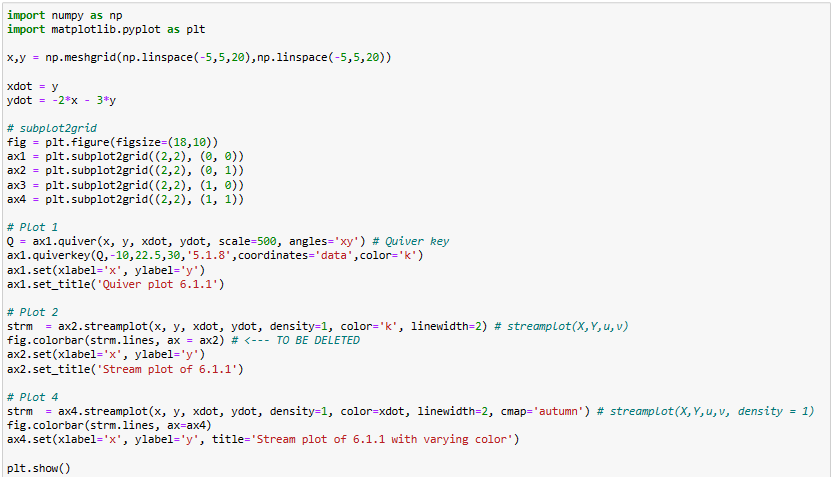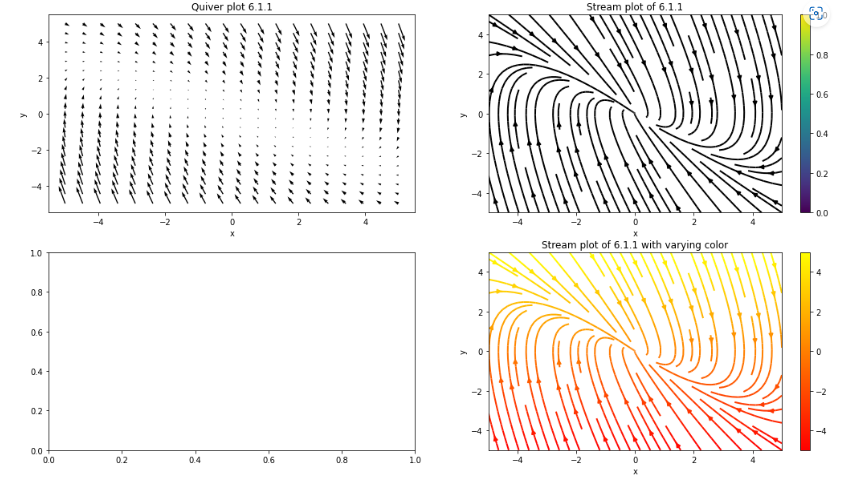# How to create Stream plot with streamlines and colors?by kanikaUpdated: Jul 12, 2023Solution Kit

A streamplot is also known as a streamlined plot. It is a data visualization technique used to depict the flow or movement of a vector field. It is useful in analyzing fluid dynamics and electromagnetic fields involving vector quantities. It visualizes the direction and magnitude by drawing curves called streamlines. Each force streamlines the path followed by a particle in the vector field. The streamline direction corresponds to the vector field direction at that point. The streamline density indicates the magnitude or strength of the vector field.

To create a streamplot, a grid of points is defined within the vector field's domain. A tangent vector is calculated at each grid point based on the vector field's values. These tangent vectors are then used to draw the streamlines. These are integrated through the vector field. It visually represents the flow patterns, convergence, divergence, and circulation.

They are useful in Flow Visualization, Field Analysis, Critical Points, and Predictive Analysis. Streamplots are designed for visualizing vector fields. They are not suitable for plotting other types of data. Streamplots visualize the vector flow or movement of vector quantities. It includes stream velocity, force, or electric field. Other techniques are:

#### Numeric Data:

Numeric data can be plotted using lines, scatter, bars, or histograms. These plots are suitable for visualizing continuous or discrete numeric data. It helps explore variable relationships, analyze distributions, or identify trends and patterns.

#### Categorical Data:

Categorical data represents discrete variables or qualitative attributes. Bar, pie charts, stacked bar plots, or categorical heatmaps help visualize categorical data.

#### Time-Series Data:

Time-series data represents data points collected at regular time intervals. Line plots, area plots, candlestick plots, and subplots help visualize time-series data.

#### Spatial Data:

Spatial data represents data associated with geographic locations or data coordinates. Maps, choropleth, scatter plots on maps, or contour plots help visualize spatial data.

#### Multivariate Data:

Multivariate data involves many variables or dimensions. Scatter, parallel coordinates, heat maps, or 3D plots help visualize and analyze multivariate data.

In a streamplot, the axes are not specific to the plot. But rather the coordinate system in which the streamlines are displayed. The axis defines the streamlined coordinates within the plot. It is while the color axis, if present, represents an extra dimension of data. The streamplot does not have separate axes beyond the coordinate system. In summary, streamplots are vital in data analysis and decision-making processes.

By representing vector fields, they unlock valuable insights. They reveal patterns and provide a comprehensive understanding of the underlying data. Leveraging streamplots empowers researchers, scientists, engineers, and decision-makers. It makes informed decisions, optimizes processes, and explores possibilities in various fields.

Here is an example of creating a Stream plot with streamlines and colors.Fig1: Preview of the Code.Fig2: Preview of the output.

### Code

In this solution, we are creating Stream plot with streamlines and colors

### Instructions

Follow the steps carefully to get the output easily.

1. Install Jupyter Notebook on your computer.
2. Open terminal and install the required libraries with following commands.
3. Install numpy - pip install numpy.
4. Install matplotlib - pip install matplotlib.
5. Copy the code using the "Copy" button above, and paste it into your IDE's Python file.
6. Run the file.

I hope you found this useful. I have added the link to dependent libraries, version information in the following sections.

I found this code snippet by searching for "Create Stream plot with streamlines and colors" in kandi. You can try any such use case!

### Dependent Libraries

numpyby numpy

Python23755Version:v1.25.0rc1The fundamental package for scientific computing with Python.

Support
Quality
Security
Reuse

numpyby numpy

Python23755Version:v1.25.0rc1License: Permissive (BSD-3-Clause)

The fundamental package for scientific computing with Python.
Support
Quality
Security
Reuse

matplotlibby matplotlib

Python17559Version:v3.7.1License: No License (null)

matplotlib: plotting with Python

Support
Quality
Security
Reuse

matplotlibby matplotlib

Python17559Version:v3.7.1License: No License

matplotlib: plotting with Python
Support
Quality
Security
Reuse

If you do not have numpy that is required to run this code, you can install it by clicking on the above link and copying the pip Install command from the Pygame page in kandi.

You can search for any dependent library on kandi like numpy

### Environment Tested

I tested this solution in the following versions. Be mindful of changes when working with other versions.

1. The solution is created in Python 3.9.6
2. The solution is tested on numpy version 1.21.4
3. The solution is tested on matplotlib version 3.5.0

Using this solution, we are able to create streamplot.

### Support

1. For any support on kandi solution kits, please use the chat
2. For further learning resources, visit the Open Weaver Community learning page.

### FAQ:

1. What is a stream plot, and how can it be created in Python using Matplotlib?

A streamplot is also known as a streamlined plot. It is a type of visualization representing a vector field's flow or movement. It displays streamlines. They show the vector field's direction and magnitude at different points. You can create a streamplot in Python using the Matplotlib and NumPy libraries. Here's a step-by-step guide:

• Import the required libraries.
• Define the grid points within the vector field's domain.
• Calculate the vector field's values at each grid point.
• Create a streamplot.
• Customize the streamplot.
• Display or save the streamplot.

2. How can one draw streamlines on an area graph to display vector flow?

To draw streamlines on an area graph to display vector flow, you can combine the streamlines. Here's a step-by-step guide:

1. Import the required libraries.
2. Define the grid points within the vector field's domain.
3. Calculate the vector field's values at each grid point.
4. Calculate the scalar field values at each grid point.
5. Create the area graph with the contour plot.
6. Create the streamlines on the area graph.
7. Customize the area graph.
8. Display or save the area graph with streamlines.

3. How do axes coordinates help define the shape of a stream plot?

The axes coordinate to help define the shape, layout, and positioning. They determine the grid points, streamline positions, aspect ratio, and coordinate system transformation. It helps shape the visual representation of the vector field's flow behavior.

4. How can field lines be represented with a streamplot?

Field lines can be represented by following certain guidelines during the plotting process. Here are some key points to ensure an accurate representation of field lines:

• Sufficient Grid Density
• Consistent Integration Method
• Vector Field Interpolation
• Streamline Integration Parameters
• Fine-tuning Streamplot Appearance

5. What is the best way to visualize vector fields with a streamplot?

The best way to visualize vector fields depends on the specific characteristics. But here are some general guidelines for visualizing vector fields using a streamplot:

Streamline Density:

Adjust the density to strike a balance between capturing details and avoiding clutter.

Color Mapping:

Choose an appropriate colormap. It enhances visibility and represents the information encoded in the color mapping.

Symbols or Markers:

Assigning distinct symbols to different vector fields or annotating streamlines with symbols. It can help differentiate and convey extra information. It facilitates a more comprehensive understanding of the data.

Contour Overlay:

Overlaying contour lines or filled contours can provide extra context.

See similar Kits and Libraries